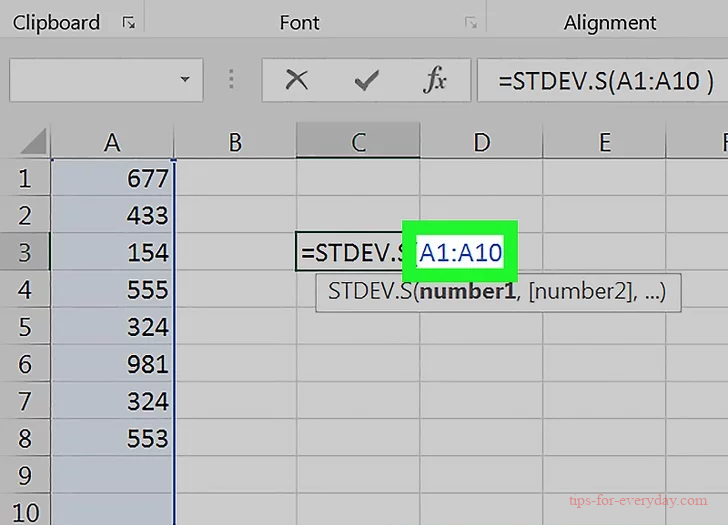Home Excel Content

# 5 Steps to Find Standard Deviation in Excel

Tips 2022-05-24 15:05:40 368

1.Enter the values you want to use. Pick a column in which you want to enter your data, then type each data value into individual cells in that column.

2.Click a blank cell. This should be the cell in which you want to display the standard deviation value. Doing so selects the cell.

3.Type in the standard deviation formula. The formula you'll type into the empty cell is =STDEV.P( ) where "P" stands for "Population". Population standard deviation takes into account all of your data points (N).4.Add your value range. In between the parentheses, type in the letter and number of the cell containing your first piece of data, type in a colon (:), and type in the letter and number of the last data cell.

For example, if you entered your data in column "A" from rows 1 through 10, you would have =STDEV.P(A1:A10) typed here.

If you just want to display the standard deviation of the value of a few scattered cells such as A1, B3, and C5, you can type the cell names separated by commas (e.g., =STDEV.P(A1,B3,C5)) instead.

5.Press Enter. This will prompt Excel to execute the formula, thus displaying the standard deviation of your selected cells in the formula's cell.